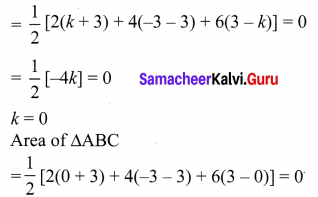## Tamilnadu Samacheer Kalvi 10th Maths Solutions Chapter 5 Coordinate Geometry Additional Questions

Question 1.
Find a relation between x and y such that the point (x, y) is equidistant from the points (7, 1) and (3, 5).
Solution:
Let P(x, y) be equidistant from the points A(7, 1) and B(3, 5).
We are given that AP = BP. So, AP2 = BP2
(x – 7)2 + (y – 1)2 =(x – 3)2 + (y – 5)2
x2 – 14x + 49 + y2 – 2y + 1 = x2 – 6x + 9 + y2 – 10y + 25
x – y = 2
Which is the required relation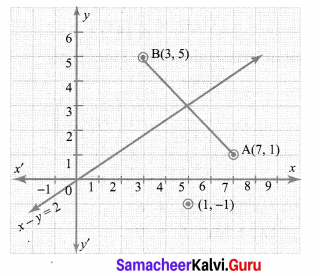Question 2.
Show that the points (1, 7), (4, 2), (-1, -1) and (-4, 4) are the vertices of a square.
Solution:
Let A(1, 7), B(4, 2), C(-1, -1) and D(-4, 4) be the given points. To prove that ABCD is a square, we have to prove that all its sides are equal and both its diagonals are equal.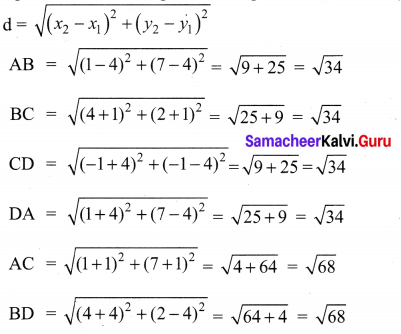Since, AB = BC = CD = DA and AC = BD, all the four sides of the quadrilateral ABCD are equal and its diagonals AC and BD are also equal. Therefore, ABCD is a square.Question 3.
If A (-5, 7), B (-4, -5), C (-1, -6) and D (4, 5) are the vertices of a quadrilateral, find the area of the quadrilateral ABCD.
Solution:
By joining B to D, you will get two triangles ABD and BCD.
Now, the area of ∆ABD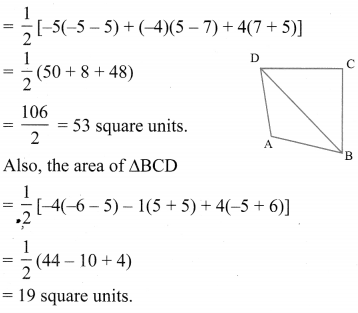So, the area of quadrilateral ABCD = 53 + 19 = 72 square units.

Question 4.
Find the coordinates of the points of trisection (i.e. points dividing in three equal parts) of the line segment joining the points A(2, -2) and B(-7, 4).
Solution:
Let P and Q be the points of trisection at AB. i.e., AP = PQ = QB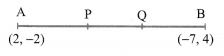Therefore, P divides AB internally in the ratio 1 : 2. Therefore, the coordinates at P, by applying the section formula, are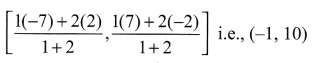Now, Q also divides AB internally in the ratio 2 : 1, so, the coordinates at Q are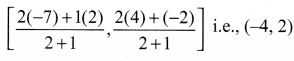Therefore, the coordinates of the points at trisection of the line segment joining A and B are (-1, 0) and (-4, 2).Question 5.
If the points A(6, 1), B(8, 2), C(9, 4) and D(P, 3) are the vertices of a parallelogram, taken in order. Find the value of P.
Solution:
We know that diagonals of a parallelogram bisect each other.
So, the coordinates at the mid-point of AC = coordinates of the mid-point of BD.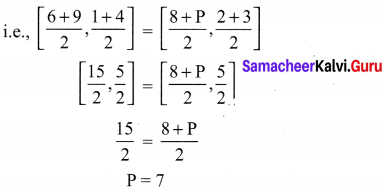Question 6.
Find the area of a triangle whose vertices are (1,-1), (-4, 6) and (-3, -5).
Solution:
The area of the triangle formed by the vertices A(1, -1), B(-4, 6) and C(-3, -5), by using the formula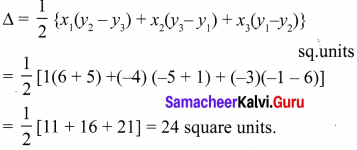So, the area of the triangle is 24 square units.

Question 7.
If A(-2, -1), B(a, 0), C(4, b) and D(1, 2) are the vertices of a parallelogram, find the values of a and b.
Solution:
We know that the diagonals of a parallelogram bisect each other. Therefore the co-ordinates of the midpoint of AC are same as the co-ordinates of the mid-point of BD. i.e.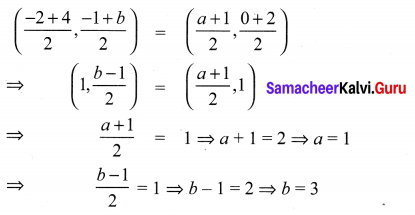Question 8.
Find the area of the quadrilateral whose vertices, taken in order, are (-3, 2), (5, 4), (7, -6) and (-5, -4).
Solution:
We have Area of the quadrilateral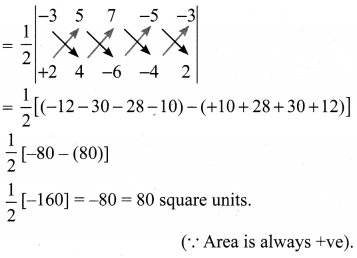Question 9.
Find the area of the triangle formed by the points P(-1.5, 3), Q(6, -2) and R(-3, 4).
Solution:
The area of the triangle formed by the given points is equal to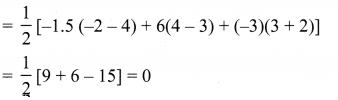Can we have a triangle of area 0 square units? What does this mean?
If the area of a triangle is 0 square units, then its vertices will be collinear.

Question 10.
Find the value of k if the pointsA(2, 3), B(4, k) and (6, -3) are collinear.
Since the given points are collinear, the area a the triangle formed by them must be 0, i.e.